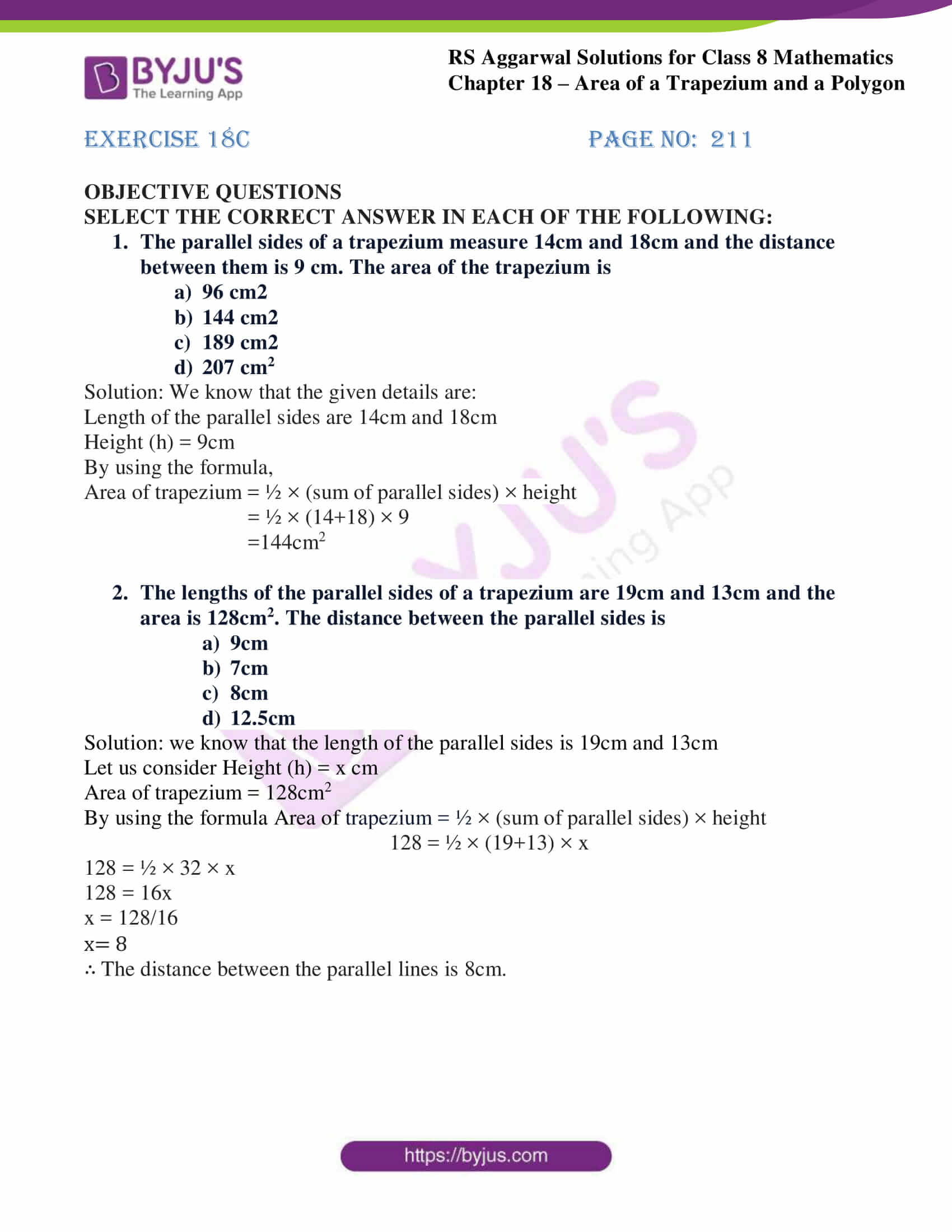# RS Aggarwal Solutions for Class 8 Maths Chapter 18 - Area of a Trapezium and a Polygon Exercise 18C

Download RS Aggarwal Solutions for Class 8 Maths Chapter 18- Exercise 18C, Area of a Trapezium and a Polygon from the links provided below. Our tutors have solved the RS Aggarwal Solutions to ensure that the students are perfect with their fundamental concepts by practicing the solutions. In Exercise 18C of RS Aggarwal Class 8 Maths, we shall brief all the topics covered in Chapter 18.

## Download PDF of RS Aggarwal Solutions for Class 8 Maths Chapter 18- Area of a Trapezium and a Polygon Exercise 18C### Access Answers to RS Aggarwal Solutions for Class 8 Maths Chapter 18- Area of a Trapezium and a Polygon Exercise 18C

SELECT THE CORRECT ANSWER IN EACH OF THE FOLLOWING:

1. The parallel sides of a trapezium measure 14cm and 18cm and the distance between them is 9 cm. The area of the trapezium is
(a) 96 cm2
(b) 144 cm2
(c) 189 cm2
(d) 207 cm2

Solution: We know that the given details are:

Length of the parallel sides are 14cm and 18cm

Height (h) = 9cm

By using the formula,

Area of trapezium = ½ × (sum of parallel sides) × height

= ½ × (14+18) × 9

=144cm2

2. The lengths of the parallel sides of a trapezium are 19cm and 13cm and the area is 128cm2. The distance between the parallel sides is
(a) 9cm
(b) 7cm
(c) 8cm
(d) 12.5cm

Solution: we know that the length of the parallel sides is 19cm and 13cm

Let us consider Height (h) = x cm

Area of trapezium = 128cm2

By using the formula Area of trapezium = ½ × (sum of parallel sides) × height

128 = ½ × (19+13) × x

128 = ½ × 32 × x

128 = 16x

x = 128/16

x= 8

∴ The distance between the parallel lines is 8cm.

### Access other Exercises of RS Aggarwal Solutions for Class 8 Maths Chapter 18- Area of a Trapezium and a Polygon

Exercise 18A Solutions 12 Questions

Exercise 18B Solutions 8 Questions

## RS Aggarwal Solutions for Class 8 Maths Chapter 18- Area of a Trapezium and a Polygon Exercise 18C

Exercise 18C of RS Aggarwal Class 8, Area of a Trapezium and a Polygon. This exercise mainly consists of Objective Questions related to the topics discussed earlier in Chapter 18.

Students are suggested to try solving the questions from RS Aggarwal book of Class 8 and then refer to these solutions to know the best way of approaching the different questions. By referring to these solutions, students develop problem-solving abilities and can tackle any type of questions easily. Thus practicing as many times as possible helps in building time management skills and also boosts the confidence level to achieve high marks.